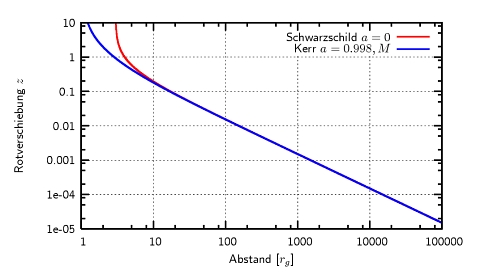# What is gravitational redshift

## Gravitational redshift

Gravitational redshift denotes one Redshift effectwho through theGravity caused: The light particles (photons) suffer a loss of radiation energy because they try to escape an attractive gravitational field.

### analogy

When we throw a ball in the air, it reaches a highest point and gravity forces it to turn back. The reason: the kinetic energy of the ball or its speed was too low for it to counter gravity in order to leave the earthly gravity field. This movement can be described very well with Newtonian gravity, e.g. to calculate the highest point on the path, the impact speed or the point of impact of the ball. If the thrown body exceeds the escape speed of the earth, it can actually leave the earth. However, this limit speed for the earth is a whopping 11.2 km / s or 40,000 km / h so that in principle only rockets can leave the earth.
Amazingly, it is the same with light, because light also loses energy when it leaves the area of ​​a gravitational field. However, this can only be correctly understood and described using the general theory of relativity. The heavy field is then replaced by one curved spacetime. The light particles move on zero geodesics. There is an analogy between light and a thrown ball: the ball loses kinetic energy, while the light particles lose radiant energy. Since a loss of radiation energy means a shift towards the red end of the spectrum, this is a redshift effect - because the cause is still gravity, this phenomenon is called Gravitational redshift.

### Extreme case of black hole

In principle, one can say that every emitter has a gravitational redshifting effect on the radiation it emits because it has a mass. With the usual small masses, however, the effect is negligible. But the effect occurs particularly drastically with compact masses like Black holes in appearance: At the event horizon they swallow every radiation because the gravitational redshift is infinitely large: this is what gives black holes their characteristic blackness.

### Gravitational redshifted lines in AGN

The effect of how much the curved spacetime of a hole pulls on the ambient radiation can be demonstrated with high-precision, astronomical measuring methods even at some distance from the hole. So show Spectral lines, which are emitted near the supermassive black hole of the active galactic core Mrk 110, measurable the gravitational redshift: This was determined by means of optical spectral lines of hydrogen and helium (Kollachny 2003) and detected by means of oxygen, nitrogen and carbon lines in the range of soft X-rays (Boller et al. 2006). The region of origin of the optical lines (the so-called broad line region, BLR) is a few to about a hundred light days away from the hole (corresponding to a hundred to a few thousand gravitational radii). The X-rays are created much closer to the hole, at around a tenth to a light day (a few tens to a hundred gravitational radii). These observations are consistent with simulation models in which the lines are given off by Kepler's rotating rings in the equatorial plane of the hole (Müller & Wold 2006). These models suggest that with modern, high-resolution telescopes, the gravitational redshift up to a distance of about 75,000 gravitational radii could be detectable! They also show that the hole rotation can only be tested with spectral lines that arise very close to the hole, e.g. relativistically broadened iron lines (Fe Kα).
The following figure shows the redshift as a function of the distance from the black hole. Not only the gravitational redshift was taken into account, but also the (longitudinal and transversal) Doppler effect. In the model, the radiation comes from a Kepler disk in the equatorial plane. As you can see the redshift is increasing z (not to be confused with the cosmological redshift!) constantly closes when you get closer to the hole. Deviations between a non-rotating Schwarzschild hole (red curve) and a rapidly rotating Kerr hole (blue curve) are only found in radii smaller than 4 gravitational radii recognizable. In other words: the emitter must at least come this close to the hole in order to test hole rotation!### From red to blue

Further details can be found in the opposite effect, the blue shift.